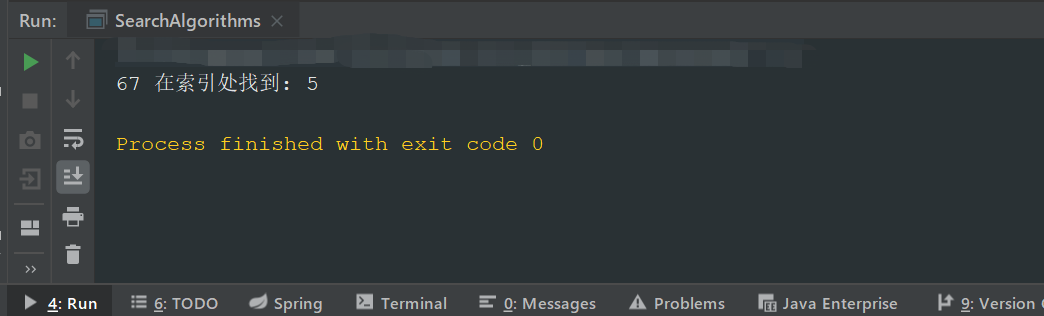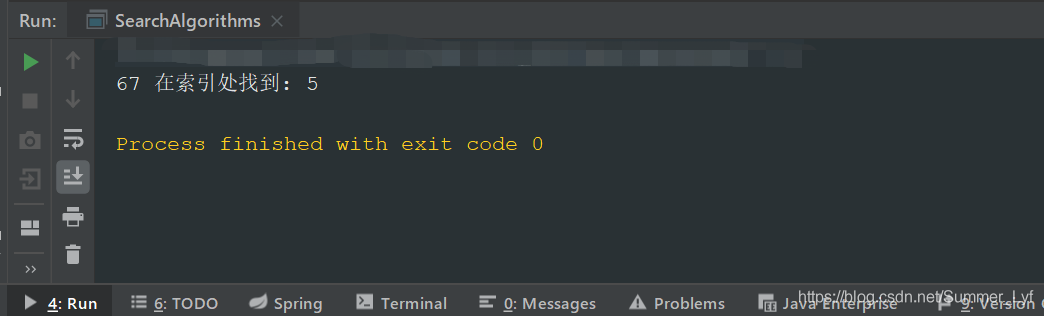• 在RFID系统中,为解决碰撞问题,必须采用一定防碰撞算法,时分多址算法中的二进制搜索算法识别率较高,是RFID系统中最常用防碰撞算法。介绍了常用防碰撞算法原理,并详细分析了二进制搜索算法的原理和实现过程,最后...
• 针对0-1 背包问题, 提出一种二进制修正和声搜索算法. 该算法修正了即兴创作过程, 对参数PAR进行动态调整, 同时提出一种随机修复机制, 有效修复不可行和声, 增强算法局部搜索. 采用一种可行和声初始化方式, 保证...
• wiki讲法是在计算机科学中，二进制搜索，也称为半间隔搜索，对数搜索，或二进制印章，是一种搜索算法，用于查找排序数组中目标值位置。二进制搜索将目标值与数组中间元素进行比较。 基本思想 当数据量很大...
目录二分查找概述基本思想二分算法写法复杂度
二分查找
概述
二分查找是一种算法，其输入是一个有序的元素列表。
wiki的讲法是在计算机科学中，二进制搜索，也称为半间隔搜索，对数搜索，或二进制印章，是一种搜索算法，用于查找排序数组中目标值的位置。二进制搜索将目标值与数组的中间元素进行比较。
基本思想

当数据量很大适宜采用该方法。
采用二分法查找时，数据需是排好序的
假设数据是按升序排序的，对于给定值key，从序列的中间位置mid开始比较:

如果当前位置arr[mid]值等于key，则查找成功；
若key小于当前位置值arr[mid]，则在数列的前半段中查找arr[low,mid-1]；
若key大于当前位置值arr[mid]，则在数列的后半段中继续查找arr[mid+1,high]，
直到找到为止，时间复杂度：O(log(n))

其中，二分法的两大特点：有序，有界。
二分算法写法

非递归二分法

int binary( int *a, int key, int n )
{
int low = 0,
high = n - 1,
mid  = (high+low)/2;

while(low < high && a[mid] != key)
{
if(a[mid] < key) {
low  = mid + 1;
} else if(a[mid] > key) {
high = mid - 1;
}
mid = (low+high)/2;
}
if(a[mid] == key) {
return mid;
}
return -1;
}


递归二分法

int recurbinary(int *a, int key, int low, int high)
{
int mid;
if(high >= low) {
mid = (high+low)/2;
if(a[mid] == key) {
return mid;
}else if(a[mid] > key) {
return recurbinary(a,key,low,mid -1);
}else {
return recurbinary(a,key,mid + 1,high);
}
}else {
return -1;
}
}

复杂度
二分法复杂度

二分法因为整个运算过程没有空间的改变,所以空间复杂度为O(1)
循环的基本次数是log2N，所以时间复杂度为O(log2N)

复杂度简介
算法复杂度分为时间复杂度和空间复杂度:
时间复杂度是指执行这个算法所需要的计算工作量；
空间复杂度是指执行这个算法所需要的内存空间。
时间和空间（即寄存器）都是计算机资源的重要体现，而算法的复杂性就是体现在运行该算法时的计算机所需的资源多少.
最坏情况下的时间复杂度称最坏时间复杂度。
一般不特别说明，讨论的时间复杂度均是最坏情况下的时间复杂度。
这样做的原因是：最坏情况下的时间复杂度是算法在任何输入实例上运行时间的上界，这就保证了算法的运行时间不会比任何更长。


展开全文二分法 数据结构
• 本期目录 一，斐波那契搜索算法简述 二，斐波那契搜索算法代码实现 三，斐波那契搜索算法总结 四，跳转搜索算法完整代码 一，斐波那契搜索算法简述 ...斐波那契搜索(Fibonacci ...与二进制搜索不同，在二进制...
本期目录

一， 斐波那契搜索算法简述

二，斐波那契搜索算法代码实现

三，斐波那契搜索算法总结

四，跳转搜索算法完整代码

一， 斐波那契搜索算法简述

斐波那契搜索(Fibonacci search) ，又称斐波那契查找，是区间中单峰函数的搜索技术。

斐波那契搜索采用分而治之的方法，其中我们按照斐波那契数列对元素进行不均等分割。此搜索需要对数组进行排序。

与二进制搜索不同，在二进制搜索中，我们将元素分成相等的两半以减小数组范围-在斐波那契搜索中，我们尝试使用加法或减法来获得较小的范围。

斐波那契数列的公式是：

此系列的前两个数字是Fibo(0) = 0和Fibo(1) = 1。因此，根据此公式，该级数看起来像是0、1、1、2、3、5、8、13、21。。。这里要注意的有趣观察是：

Fibo(N-2) 大约是1/3的 Fibo(N)

Fibo(N-1) 大约是2/3的 Fibo(N)

因此，当我们使用斐波那契数列来划分范围时，它会以与上述相同的比率进行分割。

二，斐波那契搜索算法代码实现

/**
*
* @param integers
* @param elementToSearch
* @return
*/
public static int fibonacciSearch(int[] integers, int elementToSearch) {

int fibonacciMinus2 = 0;
int fibonacciMinus1 = 1;
int fibonacciNumber = fibonacciMinus2 + fibonacciMinus1;
int arrayLength = integers.length;

while (fibonacciNumber < arrayLength) {
fibonacciMinus2 = fibonacciMinus1;
fibonacciMinus1 = fibonacciNumber;
fibonacciNumber = fibonacciMinus2 + fibonacciMinus1;
}

int offset = -1;

while (fibonacciNumber > 1) {
int i = Math.min(offset+fibonacciMinus2, arrayLength-1);

if (integers[i] < elementToSearch) {
fibonacciNumber = fibonacciMinus1;
fibonacciMinus1 = fibonacciMinus2;
fibonacciMinus2 = fibonacciNumber - fibonacciMinus1;
offset = i;
}

else if (integers[i] > elementToSearch) {
fibonacciNumber = fibonacciMinus2;
fibonacciMinus1 = fibonacciMinus1 - fibonacciMinus2;
fibonacciMinus2 = fibonacciNumber - fibonacciMinus1;
}

else return i;
}

if (fibonacciMinus1 == 1 && integers[offset+1] == elementToSearch)
return offset+1;

return -1;
}

三，斐波那契搜索算法总结

首先从找到斐波那契数列中最接近但大于数组长度的数字开始。这fibonacciNumber是在13刚好大于数组长度10时发生的。

接下来，我们比较数组的元素，并根据该比较，执行以下操作之一：

将要搜索的元素与处的元素进行比较fibonacciMinus2，如果值匹配，则返回索引。
如果elementToSearch比当前元素时，我们移动在斐波纳契数列上一步，而改变的值fibonacciNumber，fibonacciMinus1与fibonacciMinus2相应。偏移量将重置为当前索引。
如果elementToSearch比当前元素小，我们继续前进后退两步在斐波纳契数列和改变的值fibonacciNumber，fibonacciMinus1与fibonacciMinus2相应。
输出结果：时间复杂度

此搜索的最坏情况时间复杂度为O（log（N））。

空间复杂度

虽然我们需要将三个数字保存在斐波那契数列中并要搜索的元素，但我们需要四个额外的空间单位。

对空间的要求不会随着输入数组的大小而增加。因此，可以说斐波那契搜索的空间复杂度为O（1）。

当除法运算是CPU要执行操作时，将使用此搜索。二进制搜索之类的算法由于使用除法对数组进行划分，因此效果较差。

这种搜索的另一个好处是当输入数组的元素无法放入RAM中时。在这种情况下，此算法执行的局部操作范围可帮助其更快地运行。

四，跳转搜索算法完整代码

If you are interested, try it.

public class SearchAlgorithms {

/**
*
* @param integers
* @param elementToSearch
* @return
*/
public static int fibonacciSearch(int[] integers, int elementToSearch) {

int fibonacciMinus2 = 0;
int fibonacciMinus1 = 1;
int fibonacciNumber = fibonacciMinus2 + fibonacciMinus1;
int arrayLength = integers.length;

while (fibonacciNumber < arrayLength) {
fibonacciMinus2 = fibonacciMinus1;
fibonacciMinus1 = fibonacciNumber;
fibonacciNumber = fibonacciMinus2 + fibonacciMinus1;
}

int offset = -1;

while (fibonacciNumber > 1) {
int i = Math.min(offset+fibonacciMinus2, arrayLength-1);

if (integers[i] < elementToSearch) {
fibonacciNumber = fibonacciMinus1;
fibonacciMinus1 = fibonacciMinus2;
fibonacciMinus2 = fibonacciNumber - fibonacciMinus1;
offset = i;
}

else if (integers[i] > elementToSearch) {
fibonacciNumber = fibonacciMinus2;
fibonacciMinus1 = fibonacciMinus1 - fibonacciMinus2;
fibonacciMinus2 = fibonacciNumber - fibonacciMinus1;
}

else return i;
}

if (fibonacciMinus1 == 1 && integers[offset+1] == elementToSearch)
return offset+1;

return -1;
}
/**
* 打印方法
* @param elementToSearch
* @param index
*/
public static void print(int elementToSearch, int index) {
if (index == -1){
System.out.println(elementToSearch + " 未找到");
}
else {
System.out.println(elementToSearch + " 在索引处找到: " + index);
}
}
//测试一下
public static void main(String[] args) {
int index = fibonacciSearch(new int[]{3, 22, 27, 47, 57, 67, 89, 91, 95, 99}, 67);
print(67, index);
}
}


展开全文• 一， 斐波那契搜索算法简述斐波那契...与二进制搜索不同，在二进制搜索中，我们将元素分成相等两半以减小数组范围-在斐波那契搜索中，我们尝试使用加法或减法来获得较小范围。斐波那契数列公式是：Fibo(N)=F...
一， 斐波那契搜索算法简述斐波那契搜索(Fibonacci search) ，又称斐波那契查找，是区间中单峰函数的搜索技术。斐波那契搜索采用分而治之的方法，其中我们按照斐波那契数列对元素进行不均等分割。此搜索需要对数组进行排序。与二进制搜索不同，在二进制搜索中，我们将元素分成相等的两半以减小数组范围-在斐波那契搜索中，我们尝试使用加法或减法来获得较小的范围。斐波那契数列的公式是：Fibo(N)=Fibo(N-1)+Fibo(N-2)此系列的前两个数字是Fibo(0) = 0和Fibo(1) = 1。因此，根据此公式，该级数看起来像是0、1、1、2、3、5、8、13、21。。。这里要注意的有趣观察是：Fibo(N-2)大约是1/3的 Fibo(N)Fibo(N-1) 大约是2/3的 Fibo(N)因此，当我们使用斐波那契数列来划分范围时，它会以与上述相同的比率进行分割。二，斐波那契搜索算法代码实现/**** @param integers* @param elementToSearch* @return*/public static int fibonacciSearch(int[] integers, int elementToSearch) {int fibonacciMinus2 = 0;int fibonacciMinus1 = 1;int fibonacciNumber = fibonacciMinus2 + fibonacciMinus1;int arrayLength = integers.length;while (fibonacciNumber < arrayLength) {fibonacciMinus2 = fibonacciMinus1;fibonacciMinus1 = fibonacciNumber;fibonacciNumber = fibonacciMinus2 + fibonacciMinus1;}int offset = -1;while (fibonacciNumber > 1) {int i = Math.min(offset+fibonacciMinus2, arrayLength-1);if (integers[i] < elementToSearch) {fibonacciNumber = fibonacciMinus1;fibonacciMinus1 = fibonacciMinus2;fibonacciMinus2 = fibonacciNumber - fibonacciMinus1;offset = i;}else if (integers[i] > elementToSearch) {fibonacciNumber = fibonacciMinus2;fibonacciMinus1 = fibonacciMinus1 - fibonacciMinus2;fibonacciMinus2 = fibonacciNumber - fibonacciMinus1;}else return i;}if (fibonacciMinus1 == 1 && integers[offset+1] == elementToSearch)return offset+1;return -1;}三，斐波那契搜索算法总结首先从找到斐波那契数列中最接近但大于数组长度的数字开始。这fibonacciNumber是在13刚好大于数组长度10时发生的。接下来，我们比较数组的元素，并根据该比较，执行以下操作之一：将要搜索的元素与处的元素进行比较fibonacciMinus2，如果值匹配，则返回索引。如果elementToSearch比当前元素时，我们移动在斐波纳契数列上一步，而改变的值fibonacciNumber，fibonacciMinus1与fibonacciMinus2相应。偏移量将重置为当前索引。如果elementToSearch比当前元素小，我们继续前进后退两步在斐波纳契数列和改变的值fibonacciNumber，fibonacciMinus1与fibonacciMinus2相应。输出结果：时间复杂度此搜索的最坏情况时间复杂度为O(log(N))。空间复杂度虽然我们需要将三个数字保存在斐波那契数列中并要搜索的元素，但我们需要四个额外的空间单位。对空间的要求不会随着输入数组的大小而增加。因此，可以说斐波那契搜索的空间复杂度为O(1)。当除法运算是CPU要执行操作时，将使用此搜索。二进制搜索之类的算法由于使用除法对数组进行划分，因此效果较差。这种搜索的另一个好处是当输入数组的元素无法放入RAM中时。在这种情况下，此算法执行的局部操作范围可帮助其更快地运行。四，跳转搜索算法完整代码If you are interested, try it.public class SearchAlgorithms {/**** @param integers* @param elementToSearch* @return*/public static int fibonacciSearch(int[] integers, int elementToSearch) {int fibonacciMinus2 = 0;int fibonacciMinus1 = 1;int fibonacciNumber = fibonacciMinus2 + fibonacciMinus1;int arrayLength = integers.length;while (fibonacciNumber < arrayLength) {fibonacciMinus2 = fibonacciMinus1;fibonacciMinus1 = fibonacciNumber;fibonacciNumber = fibonacciMinus2 + fibonacciMinus1;}int offset = -1;while (fibonacciNumber > 1) {int i = Math.min(offset+fibonacciMinus2, arrayLength-1);if (integers[i] < elementToSearch) {fibonacciNumber = fibonacciMinus1;fibonacciMinus1 = fibonacciMinus2;fibonacciMinus2 = fibonacciNumber - fibonacciMinus1;offset = i;}else if (integers[i] > elementToSearch) {fibonacciNumber = fibonacciMinus2;fibonacciMinus1 = fibonacciMinus1 - fibonacciMinus2;fibonacciMinus2 = fibonacciNumber - fibonacciMinus1;}else return i;}if (fibonacciMinus1 == 1 && integers[offset+1] == elementToSearch)return offset+1;return -1;}/*** 打印方法* @param elementToSearch* @param index*/public static void print(int elementToSearch, int index) {if (index == -1){System.out.println(elementToSearch + " 未找到");}else {System.out.println(elementToSearch + " 在索引处找到: " + index);}}//测试一下public static void main(String[] args) {int index = fibonacciSearch(new int[]{3, 22, 27, 47, 57, 67, 89, 91, 95, 99}, 67);print(67, index);}}到此这篇关于详解Java Fibonacci Search斐波那契搜索算法代码实现的文章就介绍到这了,更多相关Java Fibonacci Search 内容请搜索脚本之家以前的文章或继续浏览下面的相关文章希望大家以后多多支持脚本之家！
展开全文java斐波那契查找
• 详细分析了典型的二进制及动态二进制防碰撞算法原理，并在此基础上提出了一种新防碰撞算法。该算法根据标签碰撞特点，充分利用已得到冲突信息，采用休眠计数方法，使搜索范围大大缩小，提高了标签识别效率...
• 防碰撞算法是RFID应用系统中关键问题之一，解决这个问题可以采用时分多路（TDMA）技术，其相 关的算法有Aloha法、时隙Aloha法、二进制搜索法、动态二进制搜索法等防碰撞算法。本文着重对Aloha算法进行 分析论证。
• 最后分别采用二进制遗传算法二进制量子遗传算法以及实数和量子比特共同编码自适应量子遗传算法对Schaffer’f6函数进行测试对比，结果表明，实数和量子比特共同编码自适应量子遗传算法无论在收敛速度还是收敛...
• 遗传算法采用选择，交叉，变异操作，在问题空间搜索最优解.经典遗传算法首先对参数进行编码，生成一定数目个体，形成初始种群其中每个个体可以是一维或多维矢量，以二进制数串表示，称为染色体.染色体每一位
﻿﻿

遗传算法(GA)作为一种经典的进化算法，自 Holland提出之后在国际上已经形成了一个比较活跃的研究领域. 人们对 GA 进行了大量的研究，提出了各种改进算法用于提高算法的收敛速度和精确性. 遗传算法采用选择，交叉，变异操作，在问题空间搜索最优解.经典遗传算法首先对参数进行编码，生成一定数目的个体，形成初始种群其中每个个体可以是一维或多维矢量，以二进制数串表示，称为染色体.染色体的每一位二进制数称为基因.根据自然界生物优胜劣汰的选择思想，算法中设计适应度函数作为评判每个个体性能优劣的标准，性能好的个体以一定概率被选择出来作为父代个体参加以后的遗传操作以生成新一代种群.算法中基本的遗传算子为染色体选择，染色体上基因杂交和基因变异.生成新一代种群后算法循环进行适应度评价、遗传操作等步骤，逐代优化，直至满足结束条件.
标准遗传算法的流程如下：

Stepl：初始化群体．

Step2：计算群体上每个个体的适应度值．

Step3：按由个体适应度值所决定的某个规则选择将进入下一代的个体．

Step4：按概率cp 进行杂交操作．

Step5：按概率mp 进行变异操作．

Step6：若满足某种停止条件，则执行 Step7，否则执行 Step2．

Step7：输出种群中适应度值最优的染色体作为问题的满意解.

一般情况下，算法的终止条件包括：1、完成了预先给定的进化代数；2、种群中的最优个体在连续若干代没有改进或平均适应度在连续若干代基本没有改进；3、所求问题最优值小于给定的阈值.

粒子群(PSO)算法是近几年来最为流行的进化算法，最早是由Kenned和Eberhart于1995年提出.PSO 算法和其他进化算法类似，也采用“群体”和“进化”的概念，通过个体间的协作与竞争，实现复杂空间中最优解的搜索．PSO 先生成初始种群，即在可行解空间中随机初始化一群粒子，每个粒子都为优化问题的一个可行解，并由目标函数为之确定一个适应值(fitness value)．PSO 不像其他进化算法那样对于个体使用进化算子，而是将每个个体看作是在n
维搜索空间中的一个没有体积和重量的粒子，每个粒子将在解空间中运动，并由一个速度决定其方向和距离.通常粒子将追随当前的最优粒子而运动，并经逐代搜索最后得到最优解．在每一代中，粒子将跟踪两个极值，一为粒子本身迄今找到的最优解 pbest ，另一为全种群迄今找到的最优解 gbest．由于认识到 PSO 在函数优化等领域所蕴含的广阔的应用前景，在 Kenned 和 Eberhart 之后很多学者都进行了这方面的研究.目前已提出了多种 PSO改进算法，并广泛应用到许多领域.

差分进化算法在 1997 年日本召开的第一届国际进化优化计算竞赛(ICEO)]表现突出，已成为进化算法(EA)的一个重要分支，很多学者开始研究 DE 算法，并取得了大量成果．2006年 CEC 国际会议将其作为专题讨论，由此可见 DE 算法已成为学者的研究热点，具有很大的发展空间.

每个个体的优劣程度根据已定义好的适应度函数来评价，这与被解决的问题有关．基本的差分进化算法实现过程如下：

Step1: 确定DE控制参数和所采用的具体策略，DE控制参数包括:种群数量、变异算子、交叉算子、最大进化代数、终止条件等．

Step2: 随机产生初始种群，进化代数 t = 1.

Step3: 对初始种群进行评价，即计算初始种群中每个个体的适应度值.

Step4: 判断是否达到终止条件或进化代数达到最大.若是，则进化终止，将此时的最佳个体作为解输出；若否，继续.

Step5：进行变异和交叉操作，对边界条件进行处理，得到临时种群.

Step6: 对临时种群进行评价，计算临时种群中每个个体的适应度值.

Step7: 进行选择操作，得到新种群.Step8: 进化代数 t = t+ 1，转步骤4.图2.1给出了算法的具体流程：

控制参数对一个全局优化算法的影响是很大的，DE的控制变量选择也有一些经验规则.

（1）种群数量.根据经验，种群数量 NP 的合理选择在5 D   10D之间，必须满足 NP ≥4以确保DE具有足够的不同的变异向量.

（2）变异算子.变异算子 F ∈ [0,2]是一个实常数因数，它决定偏差向量的放大比例.迄今为止的研究表明，小于0.4和大于1的 F 值仅偶尔有效， F = 0.5通常是一个较好的初始选择.若种群过早收敛，那么 F 或 NP 应该增加.

（3）交叉算子.交叉算子CR 是一个范围在[0,1]的实数，它是控制一个试验向量来自随机选择的变异向量而不是原来向量的概率的参数.CR 的一个较好的选择是0.1，但较大的CR 通常加速收敛，为了看是否可能获得一个快速解，可以首先尝试 CR = 0.9或 CR = 1.0.

（4）最大进化代数.它表示DE算法运行到指定的进化代数之后就停止运行，并将当前群体中的最佳个体作为所求问题的最优解输出.一般取值范围为100－200，当然根据问题的需要，可以增大最大进化代数以提高算法的求解精度，不过这样往往使得算法的运行时间过长.

（5）终止条件.除最大进化代数可作为DE的终止条件，还需要其它判定准则.一般当适应度值小于阀值时程序终止，阀值常选为610 .

上述参数中，F ，CR 与 NP 一样，在搜索过程中是常数，一般 F 和CR 影响搜索过程的收敛速度和鲁棒性，它们的优化值不仅依赖于目标函数的特性，还与 NP 有关.通常可通过在对不同值做一些试验之后利用试验和结果误差找到 F ，CR 和 NP 合适值.

遗传算法，粒子群算法，差分进化算法都属于进化算法的分枝，很多学者对这些算法进行了研究，通过不断的改进，提高了算法的性能，扩大了应用领域因此很有必要讨论这些算法的特点，针对不同应用领域和算法的适应能力，推荐不同的算法供使用将是十分有意义的工作．在文献中，作者针对广泛使用的 34 个基准函数分别对 DE，EA，PSO 进行了系列实验分析，对各种算法求解最优解问题进行了讨论.通过实验分析，DE 算法获得了最优性能，而且算法比较稳定，反复运算都能收敛到同一个解；PSO
算法收敛速度次之，但是算法不稳定，最终收敛结果容易受参数大小和初始种群的影响；EA 算法收敛速度相对比较慢，但在处理噪声问题方面，EA 能够很好的解决而 DE 算法很难处理这种噪声问题.

通过实验和文献分析，我们对遗传算法、粒子群算法、差分进化算法的一些指标分别进行分析现归纳如下：

（1）编码标准     GA 采用二进制编码，PSO、DE 都采用实数编码，近年来许多学者通过整数编码将GA 算法、PSO 算法应用与求解离散型问题，特别是 0-1 非线性优化为题，整数规划问题、混合整数规划问题，而离散的 DE 算法则研究的比较少，而采用混合编码技术的 DE 算法则研究更少.

（2）参数设置问题    DE 算法主要有两个参数要调整，而且参数设置对结果影响不太明显，因此更容易使用.相对于 GA 和 PSO 算法的参数过多，不同的参数设置对最终结果影响也比较大，因此在实际使用中，要不断调整，加大了算法的使用难度．高维问题在实际问题中，由于转化为个体的向量维数非常高，因此算法对高维问题的处理，将是很重要的.只有很好的处理高维问题，算法才能很好的应用于实际问题.

（3）高维问题
GA 对高维问题收敛速度很慢甚至很难收敛，但是 PSO 和 DE 则能很好解决.尤其是DE 算法，收敛速度很快而且结果很精确.

（4）收敛性能      对于优化问题，相对 GA，DE 和 PSO 算法收敛速度比较快，但是 PSO 容易陷入局部最优解，而且算法不稳定.

（5）应用广泛性       由于 GA 算法发明比较早，因此应用领域比较广泛，PSO 算法自从发明以来，已成为研究热点问题，这方面应用也比较多，而 DE 算法近几年才引起人们的关注而且算法性能好，因此应用领域将会增多.
原文网址：http://hanwangwang1989.blog.163.com/blog/static/168259017201431103649613/

展开全文• 路存取技术，其相关的算法有 ALOHA法、时隙 ALOHA法、二进制搜索法、动态二进制搜 索法等防碰撞算法。这几种算法在实现方式、应用效率上各有不同，本文对这几种算法进行 了分析论证。 关键词: RFID防碰撞 ...
• 我广泛使用了基于属性测试（特别是 ）来测试我的二进制搜索树和树平衡算法实现功能正确性。 能够指定方法必须具有高级属性，而无需手动生成输入数据，这有助于发现许多错误。 参见 。 这是乔治·梅森大学...
• 遗传算法采用选择，交叉，变异操作，在问题空间搜索最优解.经典遗传算法首先对参数进行编码，生成一定数目个体，形成初始种群其中每个个体可以是一维或多维矢量，以二进制数串表示，称为染色体.染色体每一位...
• 自平衡二进制搜索树。 没有 一种数据结构，用于实现数学中有向/无向图概念。 它将图存储在邻接表或矩阵中。 演算法 名称 资源 基准化 注意 定义 是 广泛使用哈希函数可产生128位哈希值。 是 包括，，，和。 ...
• 二进制编码缺点是汉明悬崖（Hamming Cliff），就是在某些相邻整数的二进制代码之间有很大汉明距离，使得遗传算法的交叉和突变都难以跨越。 格雷码（Gray Code）：在相邻整数之间汉明距离都为1。 （较好）有意义...机器学习
• 遗传算法采用选择，交叉，变异操作，在问题空间搜索最优解.经典遗传算法首先对参数进行编码，生成一定数目个体，形成初始种群其中每个个体可以是一维或多维矢量，以二进制数串表示，称为染色体.染色...
• ## 遗传算法介绍

万次阅读 2005-11-22 16:32:00
遗传算法的操作对象是一群二进制串(称为染色体、个体)，即种群。这里每一个染色体都对应问题一个解。从初始种群出发，采用基于适应值比例选择策略在当前种群中选择个体，使用杂交和变异来产生下一网络 优化 matlab
• 二进制编码缺点是汉明悬崖（Hamming Cliff），就是在某些相邻整数的二进制代码之间有很大汉明距离，使得遗传算法的交叉和突变都难以跨越。格雷码（Gray Code）：在相邻整数之间汉明距离都为1。（较好）有意义...优化
• 多目标派梯算法采用基于遗传算法动态优化策略，在多目标约束条件下搜索最优 派梯方案。根据调度方法需要采用整数编码，比采用二进制编码更能直观表示呼 梯分配情况，便于判断呼梯分配方案是否合理。为了减少...
• 采用遗传算法寻优时需要将问题候选解进行编码，即一个候选解对应一个编码，编码通常采用二进制，用“0”、“1”表示，为“1”则表示该候选解被选中，所有候选解组合在一起定义为染色体；在迭代进化过程中，通过...经验分享
• 内容涵盖了数据结构和算法基本原理，涉及数组、广义表、链表、散列表、树、图、排序搜索算法以及更多概率算法和动态规则等高级算法。此外，书中还提供了.NET框架类库中C#语言实现数据结构和算法。 　本书适合...
• 一、导论 演化算法是一类模拟自然界遗传进化规律仿生学算法，它不是一个具体的算法，而是一个算法簇。遗传算法是演化算法的一个分支，由于遗传算法的...（2）采用进制编码：直接用城市编号进行编码，染色体{...
• 本文用Python3完整实现了简单遗传算法(SGA) ...1、对问题解进行二进制编码。编码涉及精度问题，在本例中精度delta=0.0001,根据决策变量上下界确定对应此决策变量染色体基因长度（m）。假设一个决
• 4 设菲尔德大学MATLAB遗传算法工具箱（王辉） Matlab 遗传算法(Genetic Algorithm)优化工具箱是基于基本操作及终止条件、二进制和十进制相互转换等操作综合函数库。其实现步骤包括：通过输入及输出函数求出遗传...Matlab
• 348 10.2.1 邻接矩阵 348 10.2.2 邻接表 348 10.3 图遍历 350 10.3.1 采用深度优先搜索（DFS）法遍历图 350 10.3.2 采用广度优先搜索（BFS）法遍历图 359 10.4 最短路径 367 10.4.1 Dijkstra算法 367 10.4.2 ...
• 基本原理： 遗传算法是一种典型启发式算法，属于非数值算法范畴。...遗传算法的操作对象是一群二进制串（称为染色体、个体），即种群，每一个染色体都对应问题一个解。从初始种群出发，采用基于适应度函数选择MATLAB
• 内容涵盖了数据结构和算法基本原理，涉及数组、广义表、链表、散列表、树、图、排序搜索算法以及更多概率算法和动态规则等高级算法。此外，书中还提供了.NET框架类库中C#语言实现数据结构和算法。 　本书适合...
• 摘 要：本文采用SLRC400芯片，并将二进制搜索算法引入其软件编程中，按位识别碰撞，构成了矿用人员射频识别(RFID)监控系统核心。简要描述了RFID系统硬件结构和软件设计。关键词：SLRC400；RFID；二进制搜索；......